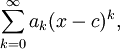# Power Series Convergence

Previous: Power Series Example

Next: Power Series Convergence Example

## The Sum May Not Converge

Our formula for the power series isFor certain values of x and ak, a power series can be infinite. Let's go back to the example we introduced earlier in this lessonThe sum of this series that tells us that the series only converges when |x| < 1 (by the divergence test). But in more general power series, there are three distinct possibilities that we can encounter.

## Three Possibilities for Convergence

Theorem: Only Three Convergence Results are Possible
A general power series seriescan only have three possibilities:

1. The series only converges when x = a
2. The series only converges when |x - a | < R, where R is some constant
3. The series converges for any real value of x

The constant R, if it exists, is called the radius of convergence. The interval of convergence of a power series, is the interval over which the series converges.

Previous: Power Series Example

Next: Power Series Convergence Example